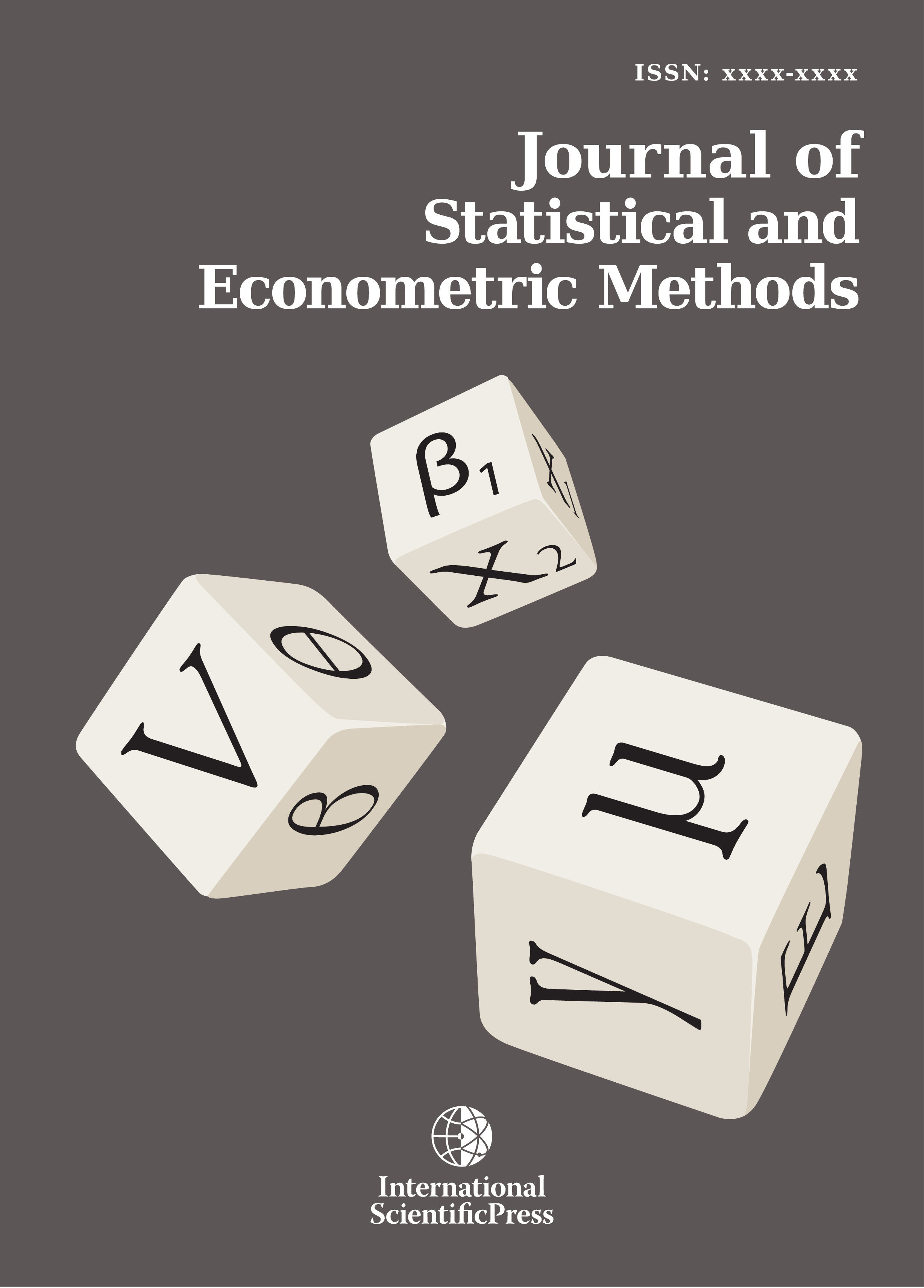# Journal of Statistical and Econometric Methods

#### A Bayesian Estimation of Stable Distributions

•[ Download ]
• Times downloaded: 1689
• Abstract

Stable distributions are a rich class of probability distributions that are widely used to model leptokurtic data. Since the probability density and distribution functions are not known in closed form, stable distributions are often specified by their characteristic functions. This paper reviews both the techniques used to compute the density functions and the methods used to estimate parameters of the stable distributions. A new Bayesian approach using Metropolis random walk chain and direct numerical integration is proposed. The performance of the method is examined by a simulation study.ISSN: 2241-0376 (Online)
2241-0384 (Print)